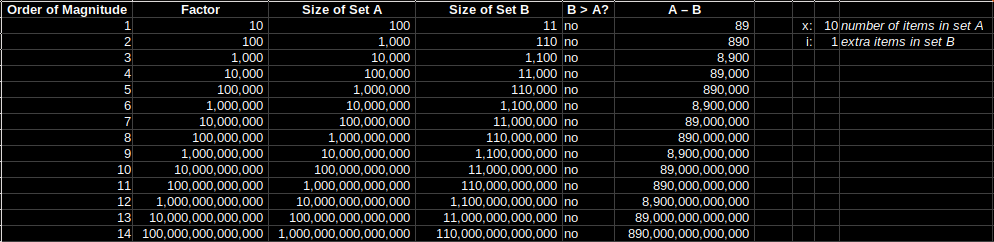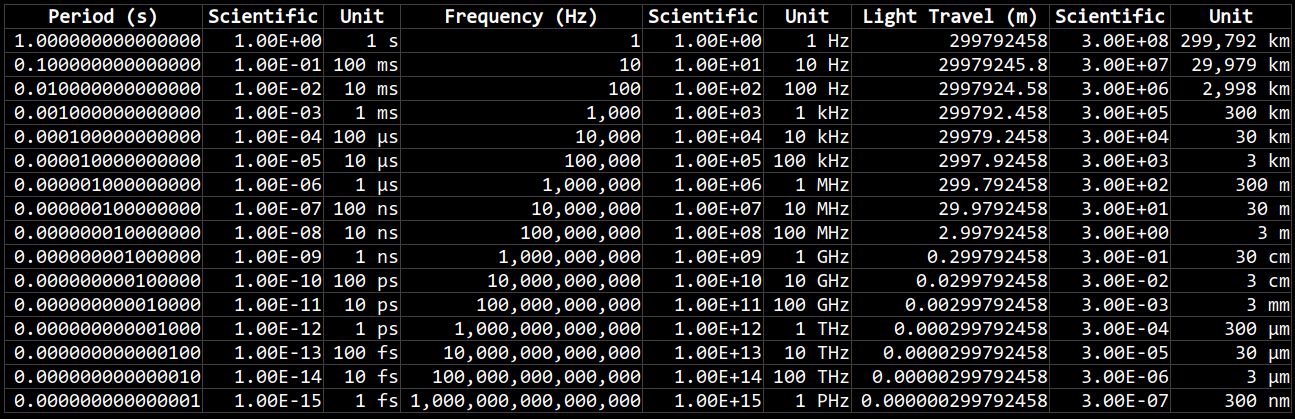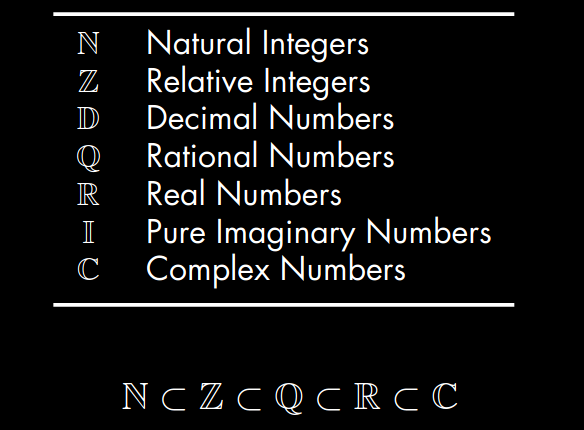# Orders of magnitude

I wanted to get some intuition for what made a bigger difference: adding an extra item to a set, or having an extra order of magnitude of items in a set. So I created this spreadsheet and discovered that an extra order of magnitude smashes an extra item in the set.In the spreadsheet the parameters are ‘x’, which is the size of the base set (called Set A), and ‘i’, which is the number of extra items to add into the second set (called Set B).

If you make x = 10 and i = 90 you break even, Set A and Set B grow at the same rate.

If you make x = 10 and i = 1 it’s no contest, Set A gets much bigger much faster.

# Period and Frequency

Oh, wait. I made it better.These charts show how period, frequency, and the speed of light are related to each other, in scientific and compact units.

Period is measured in seconds, frequency is measures in Hertz (cycles per second), and the distance of light travelled during one period is measured in meters (it is also known as the wavelength). The equation for wavelength is:

λ = c / ν

Where λ is the wavelength in meters, c is the speed of light in meters per second, and ν is the frequency of the wave in Hertz (cycles per second).

Wavelengths between 400 nanometers (nm) for violet light to 700 nm for red light are the visible component of the electromagnetic spectrum. The frequency of red light, on the low end of the visible spectrum, is about 430 terahertz (THz); whereas violet light, on the high end of the visible spectrum, is around 750 THz.

# Sets

Stolen from here.LaTeX like this:

\begin{table}[H]
\caption{Number Sets}
\label{tab:email}
\begin{tabular}{cl}
\midrule
$\mathbb{N}$ & Natural Integers \\
$\mathbb{Z}$ & Relative Integers \\
$\mathbb{D}$ & Decimal Numbers \\
$\mathbb{Q}$ & Rational Numbers \\
$\mathbb{R}$ & Real Numbers \\
$\mathbb{I}$ & Pure Imaginary Numbers \\
$\mathbb{C}$ & Complex Numbers \\
\midrule
\end{tabular}
\end{table}

$\mathbb{N} \subset \mathbb{Z} \subset \mathbb{Q} \subset \mathbb{R} \subset \mathbb{C}$


# Graphing calculator

I’m keeping my eye out for a graphing calculator. Haven’t got the money to buy one just yet, but am watching eBay to get an idea of the prices. You can see at Officeworks the choices are basically:

• HP Prime Graphing Calculator
• Casio Classpad Colour CAS Calculator fx-CP400
• Casio Colour Graphic Calculator fx-CG50 AU
• Texas Instruments Inspire Calculator CXII-CAS
• Texas Instruments Graphing Calculator TI-84 Plus CE

There’s some reviews and demos over here:

# Open Logic Project

See Open Logic Project Builds for a 1,000 page book on math fundamentals. From the home page:

The Open Logic Project is a collection of teaching materials on mathematical logic aimed at a non-mathematical audience, intended for use in advanced logic courses as taught in many philosophy departments. It is open-source: you can download the LaTeX code. It is open: you’re free to change it whichever way you like, and share your changes. It is collaborative: a team of people is working on it, using the GitHub platform, and we welcome contributions and feedback. And it is written with configurability in mind.

And if you like the Open Logic Project you might also like The Stacks project, an open source textbook and reference work on algebraic geometry.